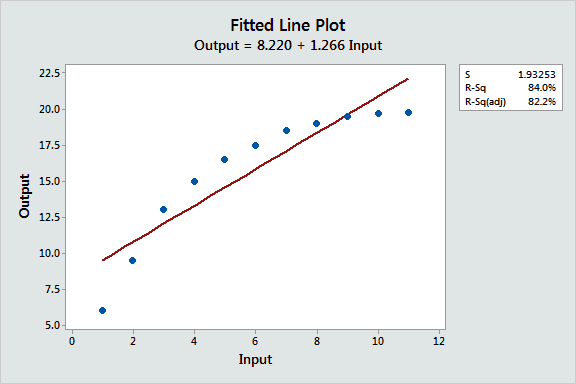We often think of a relationship between two variables as a straight line. That is, if you increase the predictor by 1 unit, the response always increases by X units. However, not all data have a linear relationship, and your model must fit the curves present in the data.

This fitted line plot shows the folly of using a line to fit a curved relationship!

How do you fit a curve to your data? Fortunately, Minitab statistical software includes a variety of curve-fitting methods in both linear regression and nonlinear regression.

To compare these methods, I’ll fit models to the somewhat tricky curve in the fitted line plot. For our purposes, we’ll assume that these data come from a low-noise physical process that has a curved function. We want to accurately predict the output given the input. Here are the data to try it yourself!

## Fitting Curves with Polynomial Terms in Linear Regression

The most common way to fit curves to the data using linear regression is to include polynomial terms, such as squared or cubed predictors.

Typically, you choose the model order by the number of bends you need in your line. Each increase in the exponent produces one more bend in the curved fitted line. It’s very rare to use more than a cubic term.

Model Order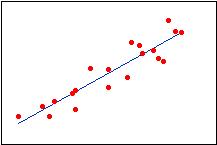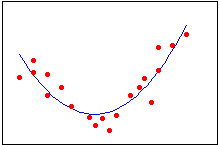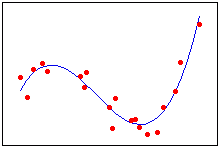The graph of our data appears to have one bend, so let’s try fitting a quadratic linear model using Stat > Fitted Line Plot.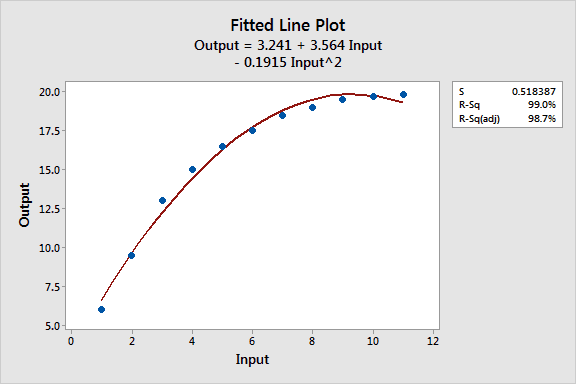While the R-squared is high, the fitted line plot shows that the regression line systematically over- and under-predicts the data at different points in the curve. This shows that you can’t always trust a high R-squared.

Let’s see if we can do better.

## Fitting Curves with Reciprocal Terms in Linear Regression

If your response data descends down to a floor, or ascends up to a ceiling as the input increases (e.g., approaches an asymptote), you can fit this type of curve in linear regression by including the reciprocal (1/X) of one more predictor variables in the model. More generally, you want to use this form when the size of the effect for a predictor variable decreases as its value increases.

Because the slope is a function of 1/X, the slope gets flatter as X increases. For this type of model, X can never equal 0 because you can’t divide by zero.

Looking at our data, it does appear to be flattening out and approaching an asymptote somewhere around 20.

I used Calc > Calculator in Minitab to create a 1/Input column (InvInput). Let’s see how that works! I fit it with both a linear (top) and quadratic model (bottom).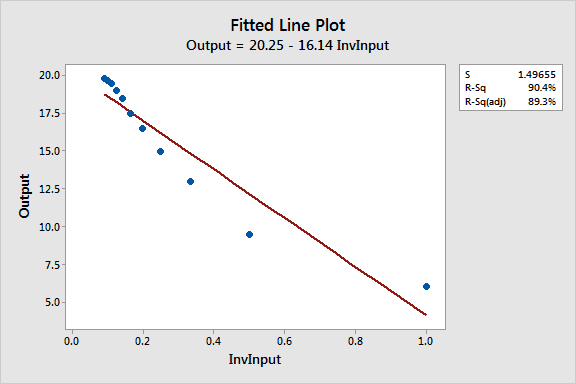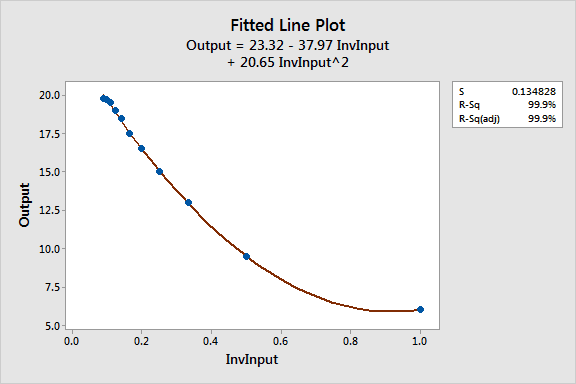For this particular example, the quadratic reciprocal model fits the data much better. The fitted line plots change the x-axis to 1/Input, so it’s hard to see the natural curvature of the data.

In the scatterplot below, I used the equations to plot fitted points for both models in the natural scale. The green data points clearly fall closer to the quadratic line.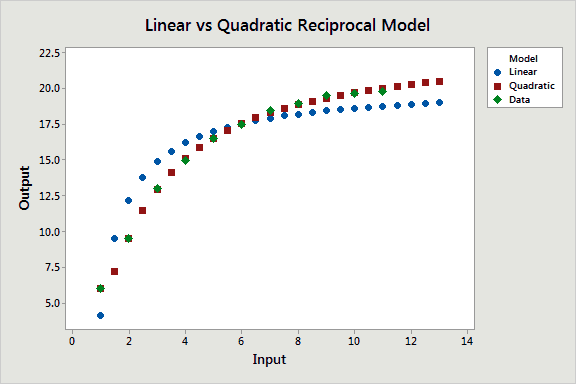Compared to the quadratic model, the reciprocal model with the quadratic term has a lower S value (good), higher R-squared (good), and it doesn’t exhibit the biased predictions. So far, this is our best model.

## Transforming the Variables with Log Functions in Linear Regression

A log transformation is a relatively common method that allows linear regression to perform curve fitting that would otherwise only be possible in nonlinear regression.

For example, the nonlinear function:

Y=eB0X1B1X2B2

can be expressed in linear form of:

Ln Y = B0 + B1lnX1 + B2lnX2

You can take the log of both sides of the equation, like above, which is called the double-log form. Or, you can take the log of just one side, known as the semi-log form. If you take the logs on the predictor side, it can be for all or just some of the predictors.

Log functional forms can be quite powerful, but there are too many combinations to get into detail in this overview. The choice of double-log versus semi-log (for either the response or predictors) depends on the specifics of your data and subject area knowledge. In other words, if you go this route, you’ll need to do some research.

Let’s get back to our example. For data where the curve flattens out as the predictor increases, a semi-log model of the relevant predictor(s) can fit. Let’s try it!

Minitab’s fitted line plot conveniently has the option to log-transform one or both sides of the model. So I’ve transformed just the predictor variable in the fitted line plot below.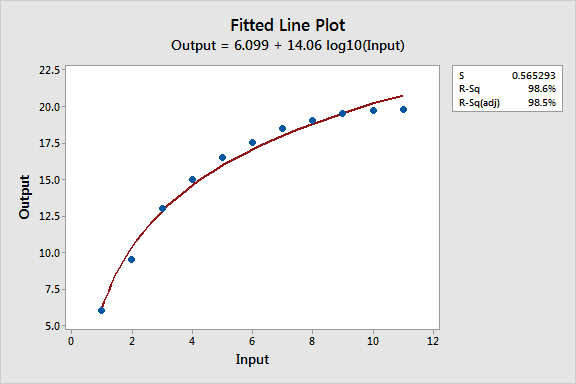Visually, we can see that the semi-log model systematically over and under-predicts the data at different points in the curve, just like quadratic model. The S and R-squared values are also virtually identical to that model.

So far, the linear model with the reciprocal terms still provides the best fit for our curved data.

## Fitting Curves with Nonlinear Regression

Nonlinear regression can be a powerful alternative to linear regression because it provides the most flexible curve-fitting functionality. The trick is to find the nonlinear function that best fits the specific curve in your data. Fortunately, Minitab provides tools to make that easier.

In the Nonlinear Regression dialog (Stat > Regression > Nonlinear Regression), enter Output for Response. Next, click Use Catalog to choose from the nonlinear functions that Minitab supplies.

We know that our data approaches an asymptote, so we can click on the two Asymptotic Regression functions. The concave version matches our data more closely. Choose that function and click OK.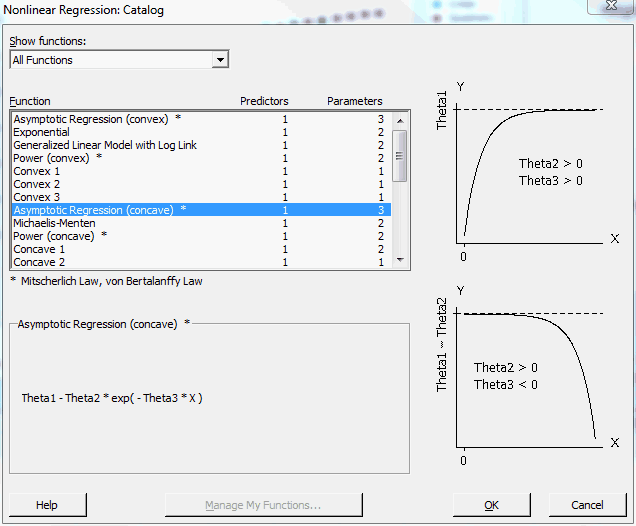Next, Minitab displays a dialog where we choose our predictor.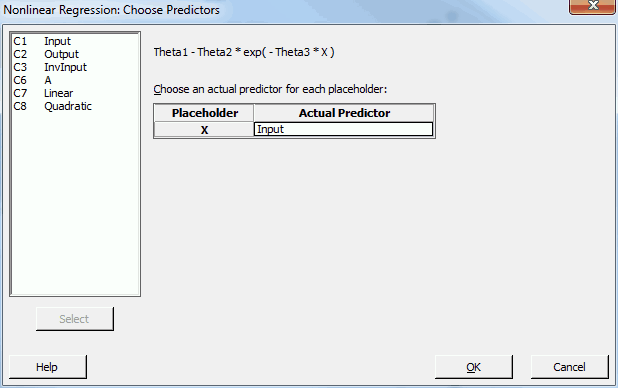Enter Input, click OK, and we’re back at the main dialog.

If we click OK in the main dialog, Minitab displays the following dialog: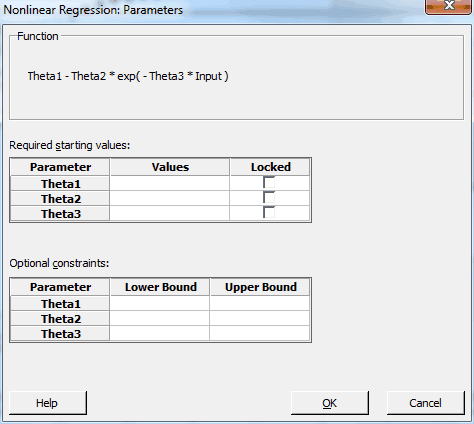Unlike linear regression, nonlinear regression uses an algorithm to find the best fit step-by-step. We need to supply the starting values for each parameter in the function. Shoot, I don’t have any idea! Fortunately, Minitab makes it easy.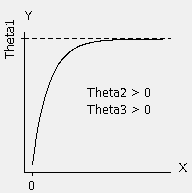Let’s look back at the function we chose. The picture makes it easier!

Notice that Theta1 is the asymptote, or the ceiling, that our data approaches. Judging by the initial scatterplot, that’s about 20 for our data. For a case like ours, where the response approaches a ceiling as the predictor increases, Theta2 > 0 and Theta3 > 0.

Consequently, I’ll enter the following in the dialog:

• Theta1: 20
• Theta2: 1
• Theta3: 1

After we enter these values, we go back to the main dialog, click OK, and voila!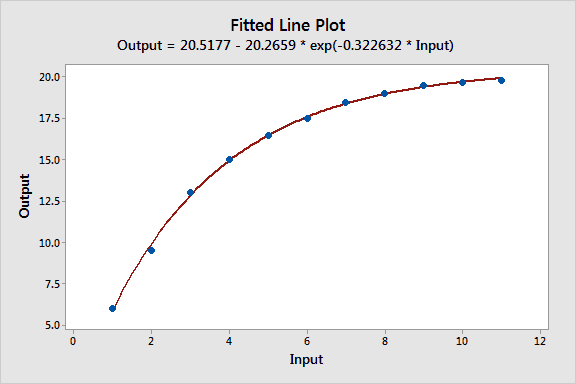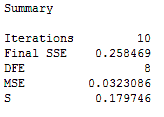It’s impossible to calculate R-squared for nonlinear regression, but the S value for the nonlinear model (0.179746) is nearly as small as that for the reciprocal model (0.134828). You want a small S because it means the data points fall closer to the curved fitted line. The nonlinear model also doesn’t have a systematic bias.

## Comparing the Curve-Fitting Effectiveness of the Different Models

 Model R-squared S Biased fits Reciprocal - Quadratic 99.9 0.134828 No Nonlinear N/A 0.179746 No Quadratic 99.0 0.518387 Yes Semi-Log 98.6 0.565293 Yes Reciprocal - Linear 90.4 1.49655 Yes Linear 84.0 1.93253 Yes

The linear model with the quadratic reciprocal term and the nonlinear model both beat the other models. These top two models produce equally good predictions for the curved relationship. However, the linear regression model with the reciprocal terms also produces p-values for the predictors (all significant) and an R-squared (99.9%), none of which you can get for a nonlinear regression model.

For this example, these extra statistics can be handy for reporting, even though the nonlinear results are equally valid. However, in cases where the nonlinear model provides the best fit, you should go with the better fit.

What is the difference between linear and nonlinear regression equations?

## Closing Thoughts

If you have a difficult curve to fit, finding the correct model may seem like an overwhelming task. However, after all the effort to collect the data, it’s worth the effort to find the best fit possible.

When specifying any model, you should let theory and subject-area knowledge guide you. Some areas have standard practices and functions to model the data.

While you want a good fit, you don’t want to artificially inflate the R-squared with an overly complicated model. Be aware that:

If you're learning about regression, read my regression tutorial!